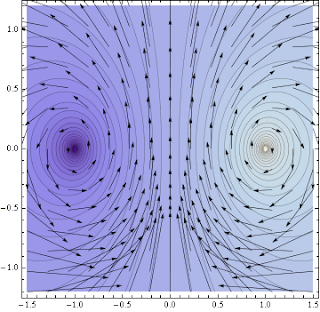## Tuesday, December 11, 2007

### Resistance is real

Following Clifford's hint I have had my nerdness tested. The version 2 result is here:It seems a science/math post is long overdue. So here it is: Resistance of a wave guide/coax cable. Readers of the Feynman Lectures know that you can model a coax cable by an infinite sequence of capacitors and inductance likethisTo compute the asymptotic (complex) resistance of this infinite circuit Feynamn instructs you look at a single iteration and to summarise the rest in some black box with resistance R.The new resistance between the terminals is then easily computed (when driven with frequency w):Now you argue that an infinite chain should not change its resistance if one link is added and thus R=R'. This quadratic equation is solved asThe final thing is to remember that the chain of L's and C's is a discrete version of a continuous chain and thus one should take both L and C to zero while keeping their ratio fixed. We end up withNote that in this limit the frequency w dropped out. So far the Feynman lectures.

But there is one curious thing: Although we have been adding only capacitors and inductances which have purely imaginary resistances and no Ohmic (real) resistance, nevertheless, the limit is real!

How can this be true? When you think about the physics you should be even more irritated: Neither capacitors nor inductances do any work and only Ohmic resistance produces heat. By adding together elements that do not produce heat. After meditating this fact for a while one settle with the explanation that one might say that the energy is carried down the infinite circuit and never returns and thus is gone and might be considered heat. But this is not really convincing.

So we should better study the maths to some more detail. What we have done was to consider a sequence of resistances and computed the possible fixed points of this iteration. If the resistance converges it certainly will converge to a fixed point. But who tells you it really converges?

So, let's again add one infinitessimal bit of chain. Let us use new variables z and x such thatThus z is the fixed point resistance we computed above and x is the thing which we take to 0 (and thus we can work at O(x)). We do the above calculation again and find that the change in resistance isWe can view i(z^2-R^2)/z as a vector field in the complex R planeWe can see (either from the formula or the plot) that for purely imaginary R we will always stay on the imaginary axis and flow to complex infinity! Without Ohmic resistance we will not flow towards the fixed points.

But even if we start off slightly off the imaginary axis we do not spiral in to one fixed point as one might have thought: The vector field is holomorphic and thus Hamiltonian. Therefore there is a conserved quantity (although right now I am too tired to compute it).

Well, I thought I might not be too tired, got confused for two entire hours and with the help of Enrico (who suggested separation of variables) and Jan found the conserved quantity to be

UPDATE: When I wrote this last night I was too tired to correctly compute the real part of 1-R^2. Thus I got the real components of the vector field wrong and this explains why I had such trouble to find the correct conserved quantity. After one short night of sleep I noticed my error and indeed the conserved quantity I had calculated earlier was correct,(setting w=z=1) but the vector field was wrong (plots are corrected as well). See for example